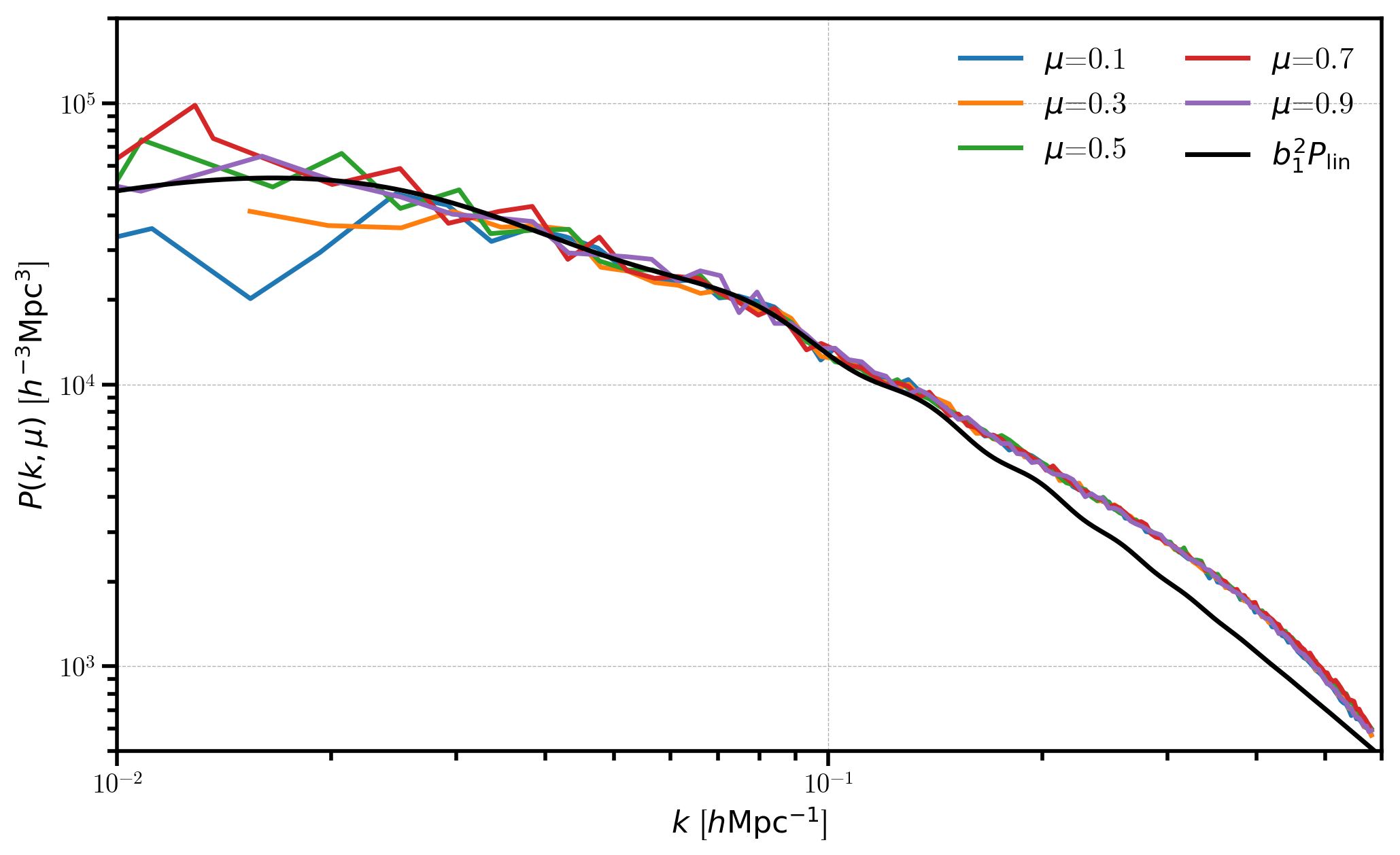# Log-normal Mocks¶

In this notebook, we generate a LogNormalCatalog at $$z=0.55$$ using the Planck 2015 cosmology parameters and the Eisenstein-Hu linear power spectrum fitting formula. We then plot the power spectra in both real and redshift space and compare to the input linear power spectrum.

In :

%matplotlib inline
%config InlineBackend.figure_format = 'retina'

In :

from nbodykit.lab import *
from nbodykit import style, setup_logging

import matplotlib.pyplot as plt
plt.style.use(style.notebook)

In :

setup_logging() # turn on logging to screen


## Initalizing the Log-normal Mock¶

Here, we generate a mock catalog of biased objects ($$b_1 = 2$$ ) at a redshif $$z=0.55$$. We use the Planck 2015 cosmology and the Eisenstein-Hu linear power spectrum fitting formula.

We generate the catalog in a box of side length $$L = 1380 \ \mathrm{Mpc}/h$$ with a constant number density $$\bar{n} = 3 \times 10^{-3} \ h^{3} \mathrm{Mpc}^{-3}$$.

In :

redshift = 0.55
cosmo = cosmology.Planck15
Plin = cosmology.LinearPower(cosmo, redshift, transfer='EisensteinHu')
b1 = 2.0

cat = LogNormalCatalog(Plin=Plin, nbar=3e-3, BoxSize=1380., Nmesh=256, bias=b1, seed=42)


## Real-space Power Spectrum¶

In this section, we compute and plot the power spectrum, $$P(k,\mu)$$, in real space (no redshift distortions) using five $$\mu$$ bins.

In :

# convert the catalog to the mesh, with CIC interpolation
real_mesh = cat.to_mesh(compensated=True, window='cic', position='Position')

# compute the 2d P(k,mu) power, with 5 mu bins
r = FFTPower(real_mesh, mode='2d', Nmu=5)
pkmu = r.power

[ 000017.54 ]   0: 10-30 20:51  CatalogMesh     INFO     painted 7887677 out of 7887677 objects to mesh
[ 000017.54 ]   0: 10-30 20:51  CatalogMesh     INFO     mean particles per cell is 0.470142
[ 000017.54 ]   0: 10-30 20:51  CatalogMesh     INFO     sum is 7.88768e+06
[ 000017.55 ]   0: 10-30 20:51  CatalogMesh     INFO     normalized the convention to 1 + delta
[ 000017.91 ]   0: 10-30 20:51  CatalogMesh     INFO     field: (LogNormalCatalog(seed=42, bias=2) as CatalogMesh) painting done

In :

# plot each mu bin
for i in range(pkmu.shape):
Pk = pkmu[:,i]
label = r'$\mu$=%.1f' %pkmu.coords['mu'][i]
plt.loglog(Pk['k'], Pk['power'].real - Pk.attrs['shotnoise'], label=label)

# plot the biased linear power spectrum
k = numpy.logspace(-2, 0, 512)
plt.loglog(k, b1**2 * Plin(k), c='k', label=r'$b_1^2 P_\mathrm{lin}$')

# add a legend and axes labels
plt.legend(loc=0, ncol=2, fontsize=16)
plt.xlabel(r"$k$ [$h \mathrm{Mpc}^{-1}$]")
plt.ylabel(r"$P(k, \mu)$ [$h^{-3} \mathrm{Mpc}^3$]")
plt.xlim(0.01, 0.6)
plt.ylim(500, 2e5)

Out:

(500, 200000.0)In this figure, we see the power spectrum in real-space is independent of $$\mu$$, as expected. At low $$k$$, the measured power agrees with the biased linear power $$b_1^2 P_\mathrm{lin}$$. But at high $$k$$, we see the effects of applying the Zel’dovich approximation to the density field, mirroring the effects of nonlinear evolution.

## Redshift-space Power Spectrum¶

In this section, we compute and plot the power spectrum, $$P(k,\mu)$$, in redshift space and see the effects of redshift-space distortions on the 2D power spectrum.

In :

def kaiser_pkmu(k, mu):
"""
Returns the Kaiser linear P(k,mu) in redshift space

.. math::

P(k,mu) = (1 + f/b_1 mu^2)^2 b_1^2 P_\mathrm{lin}(k)
"""
beta = cosmo.scale_independent_growth_rate(redshift) / b1
return (1 + beta*mu**2)**2 * b1**2 * Plin(k)


Here, we add redshift space distortions (RSD) along the $$z$$-axis of the box. We use the VelocityOffset column which is equal to the Velocity column re-normalized properly for RSD.

In :

LOS = [0,0,1] # the z-axis

# add RSD to the Position
cat['RSDPosition'] = cat['Position'] + cat['VelocityOffset'] * LOS

In :

# from catalog to mesh
rsd_mesh = cat.to_mesh(compensated=True, window='cic', position='RSDPosition')

# compute the 2D power
r = FFTPower(rsd_mesh, mode='2d', Nmu=5, los=LOS)
pkmu = r.power

# plot each mu power bin, normalized by the expected Kaiser power
for i in range(pkmu.shape):
Pk = pkmu[:,i]
mu = pkmu.coords['mu'][i]
label = r'$\mu$=%.1f' %mu

P = Pk['power'].real-Pk.attrs['shotnoise']
plt.plot(Pk['k'], P / kaiser_pkmu(Pk['k'], mu), label=label)

# add a legend and axis labels
plt.legend(loc=0, ncol=2)
plt.xlabel(r"$k$ [$h \ \mathrm{Mpc}^{-1}$]")
plt.ylabel(r"$P(k, \mu) / P^\mathrm{Kaiser}(k,\mu)$")
plt.xlim(0.01, 0.6)
plt.ylim(0, 2.5)

[ 000026.10 ]   0: 10-30 20:51  CatalogMesh     INFO     painted 7887677 out of 7887677 objects to mesh
[ 000026.10 ]   0: 10-30 20:51  CatalogMesh     INFO     mean particles per cell is 0.470142
[ 000026.10 ]   0: 10-30 20:51  CatalogMesh     INFO     sum is 7.88768e+06
[ 000026.10 ]   0: 10-30 20:51  CatalogMesh     INFO     normalized the convention to 1 + delta
[ 000026.46 ]   0: 10-30 20:51  CatalogMesh     INFO     field: (LogNormalCatalog(seed=42, bias=2) as CatalogMesh) painting done
/Users/nhand/anaconda/envs/py36/lib/python3.6/site-packages/ipykernel_launcher.py:15: RuntimeWarning: divide by zero encountered in true_divide
from ipykernel import kernelapp as app

Out:

(0, 2.5)In this figure, we clearly see the effects of RSD, which causes the higher $$\mu$$ bins to be damped at high $$k$$, relative to the lower $$\mu$$ bins. Also, the measured power agrees with the expected Kaiser formula for linear RSD at low $$k$$ (where linear theory holds): $$P^\mathrm{Kaiser} = (1 + f/b_1 \mu^2)^2 b_1^2 P_\mathrm{lin}(k)$$.